# Joel Shaffer's Marching Cubes

## Marching Cube Algorithm Overview

• Read in all the data such that for any given point (x, y, z) there is an associated value
• Iterate over each point and construct a cube
• For the cube at each point check if the associated value is less than the specified ISO value
• If the value is less than the ISO, the cube intersects the surface at that point and a bit mask should be set for the specified vertex
• Now that each vertex is determined as either inside or outside the surface, determine where along the edges the surface intersects
• Edge intersections are determined using a linear interpolation between the points, utilizing the associated data and the ISO level
• Using the triangle table and the vertex bit mask set the coordinates for a triangle
• Calculate a surface normal for each triangle
• Once all triangle data has been determined, send the data to the vertext and fragment shaders for rasterization

## My Code

• Aggregate data from 100+ image files into a single data source
• Create a 3D array representing the data
• Perform marching cubes algorithm to determine triangles
• Calculate surface normals for each triangle
• Send triangles and normal data to vertex/fragment shader (Gourad)
• Use keyboard commands (WASD) and mouse (rotation) to move around the model

## Triangle / Cube Intersections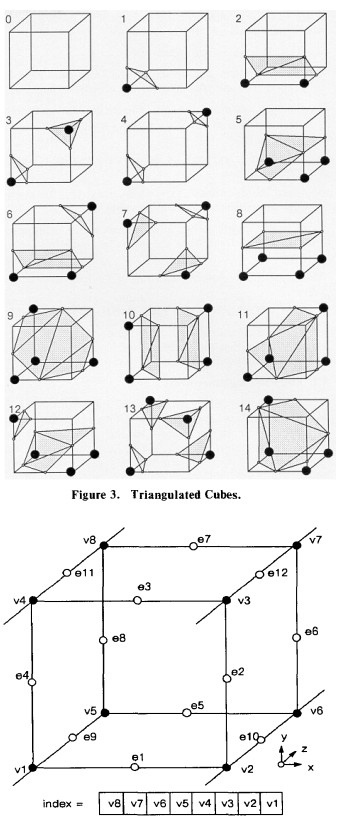## Original Stanford Images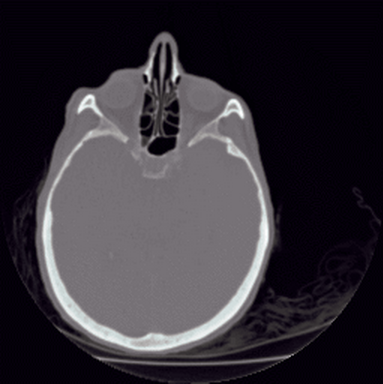## Examples of my volume visualizations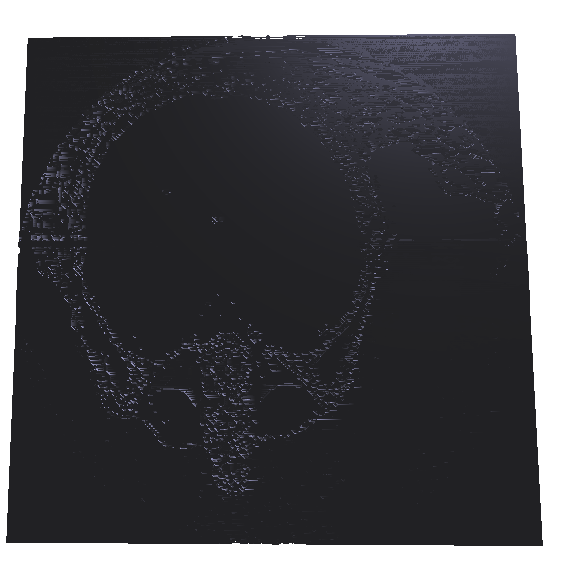### ISO 20 Surface Normals - 6,851,966 Triangles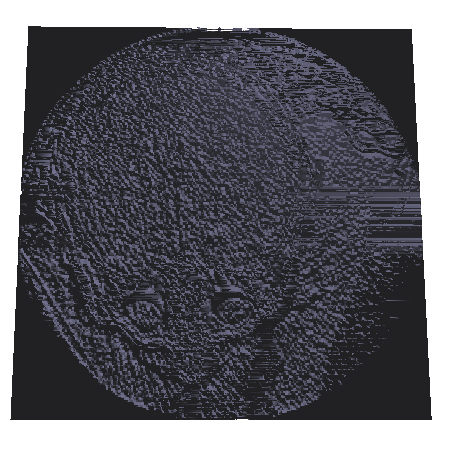### ISO 20 Vertex Normals- 6,851,966 Triangles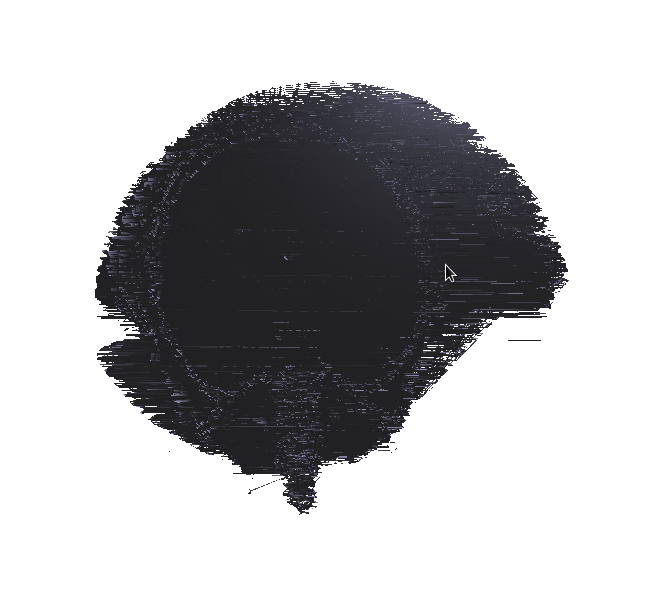### ISO 80 - 2,617,506 Triangles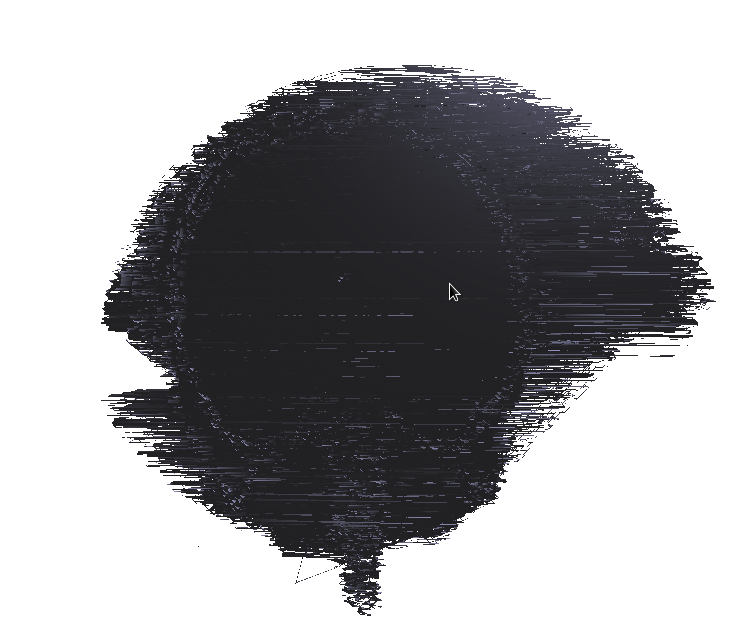### ISO 100 - 2,103,652 Triangles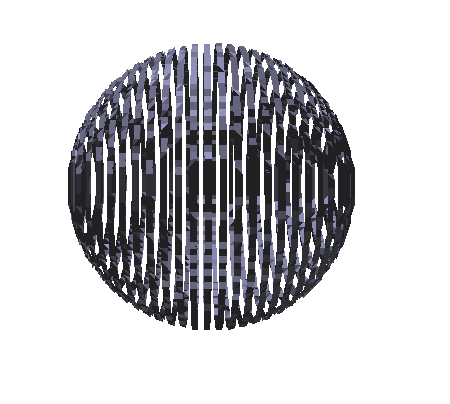### Side view of sphere slices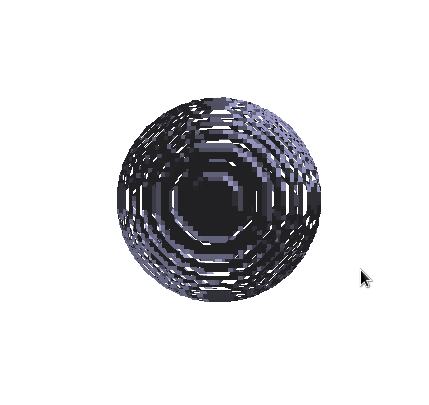### Entire Sphere - 23480 Triangles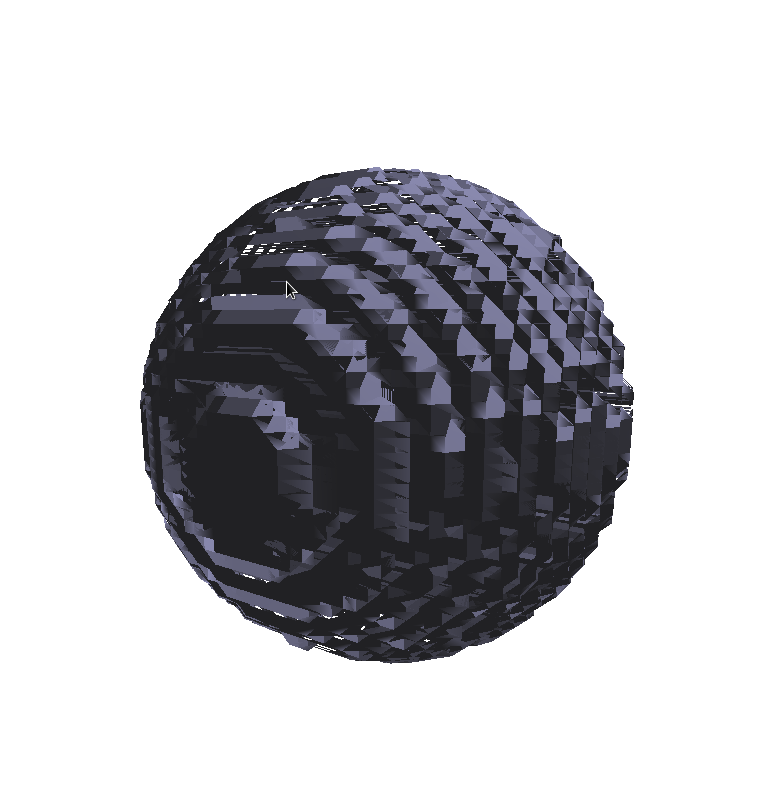### Zoomed in Sphere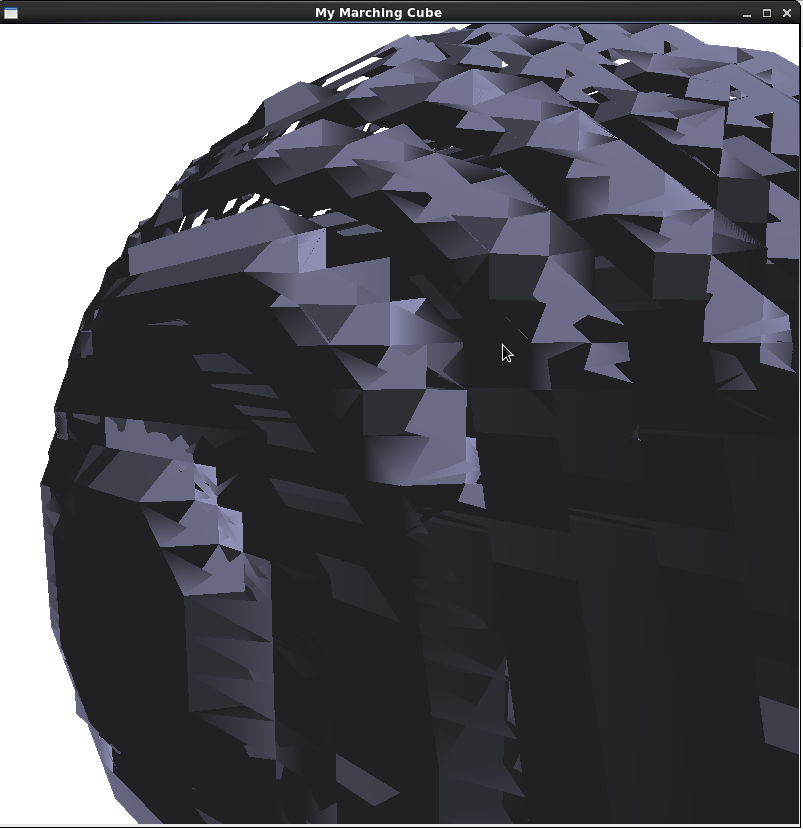### Individual triangles on Sphere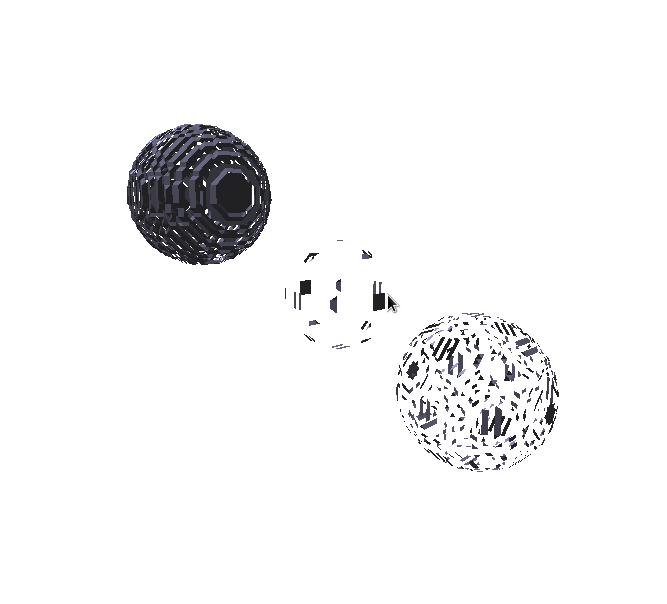### Multiple spheres of varying data quality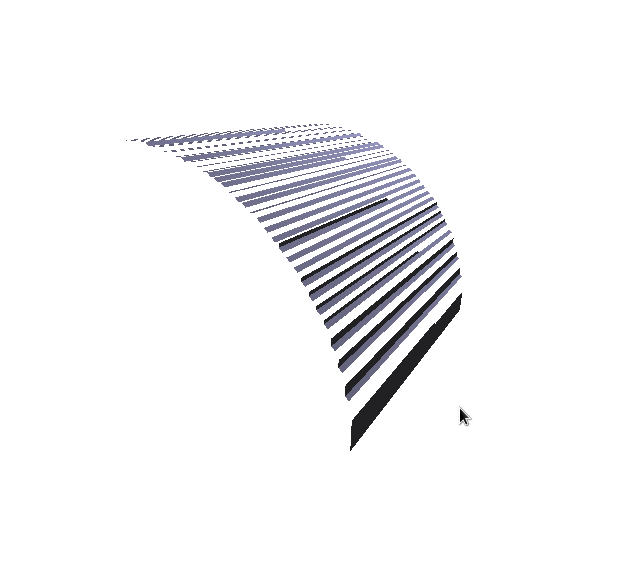## References

• Paul Bourke - Polygonising a scalar field - Triangle and Edge tables used from this source - here
• William E. Lorensen - MARCHING CUBES: A HIGH RESOLUTION 3D SURFACE CONSTRUCTION ALGORITHM - here
• Ostfold University College - Marching Cubes - here
• Stanford University Volume Data Archive - CTHead data and MRbrain data - here